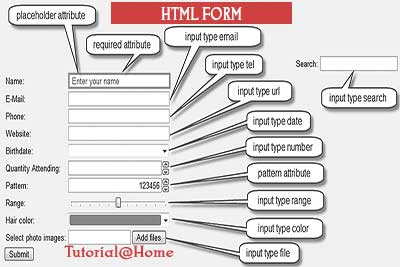HTML 5 Forms : Search Box & Range
07-05-2017    3018 times# Search Box & Range

## Search Box

In HTML5 provide another input type called search. By this input type we can define a textbox as a search box. But this control cannot produce the same result in all the leading Web Browser. The Syntax is given below

<input id="mysearchbox" type="search" />

Output:

It is like a normal textbox, but when a user writes something on it the a blue cross sign(×) appears at the right hand side of the textbox. If we click on the (×) sign, then the text of the textbox will deleted.

Note: This control gives a cute appearance of the search box, But It is not automatically connected to the search engine. Only Safari (tested with Safari 5) and Google Chrome support this input type does that.

## Input Type : Range

Range input type introduces in HTML5. It is actually shown a Slider control. It is used to control a number within a range. In the early days, if we want to use a slider control, then we must take help from the Java Applet or Javascript or flash. But HTML5.0 save lot of time and thousands of byte from programmer code.

The code can be as simple as :-

<input id="test" type="range"/>

The following attribute is used for Range type input.

 Attribute Descriptions min Minimum value of the range. Default minimum value is 0. max Maximum value of the range. Default maximum value is 100. step This attribute uses an integer number only. But if the minimum value of the control is a non integer, then the step value may also be a non integer.

### Now we are try to understand by Demos.

<!DOCTYPE html>

<html>

<title>Range Control Demo</title>

<script>

function printValue(sliderID, textbox) {

var x = document.getElementById(textbox);

var y = document.getElementById(sliderID);

x.value = y.value;

}

window.onload = function() { printValue('slider1', 't1'); printValue('slider2', 't2'); printValue('slider3', 't3'); }

</script>

<body>

<h3>Demo 1</h3>

<input id="slider1" type="range" min="150" max="650" step="5" onchange="printValue('slider1','t1')"/>

<input id="t1" type="text"><br><br><br>

<h3>Demo 2</h3>

<input id="slider2" type ="range" min ="-200" max="500" step ="20" value ="-200" onchange="printValue('slider2','t2')"/>

<input id="t2" type="text"><br><br><br>

<h3>Demo 3</h3>

<input id="slider3" type ="range" min ="-3.2" max="3.1" step ="0.1" value="2.5" onchange="printValue('slider3','t3')"/>

<input id="t3" type="text"><br><br><br>

</body></html>